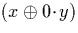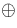Next: Useful Properties Up: Algorithm Design Previous: Algorithm Design

## Considerations

When designing algorithms for arithmetic and related operations, there are a number of considerations:

Convergence:
It must be possible to determine a new output digit after examining a finite number of digits from the input streams. If we need to examine a potentially infinite number of input digits in order to determine an output digit, then we do not have an algorithm. There are a number of subtle ways in which such a situation can arise, and care must be taken.
Correctness:
The algorithm must compute the correct result.

Efficiency:
The efficiency of the algorithm is also a consideration. Ideally we would like an algorithm that computes the result using as few digits of input as possible and which does not require manipulation of excessively large data structures. In addition, we would rather the algorithm did not branch excessively. For example, the operation, whereis the average operation, if näively implemented, would require the evaluation of each digit of y, a multiplication by zero on each digit, and a stream average operation. In fact this could be more efficiently implemented by using the `cons' algorithm to prefix a zero to the stream representing x (ie. return (0::x)--see section 3.6.2). Different algorithms for the same operation can have very different levels of performance. The issue of the efficiency of the algorithms is discussed in more detail in chapter 7.Next: Useful Properties Up: Algorithm Design Previous: Algorithm Design
Martin Escardo
5/11/2000